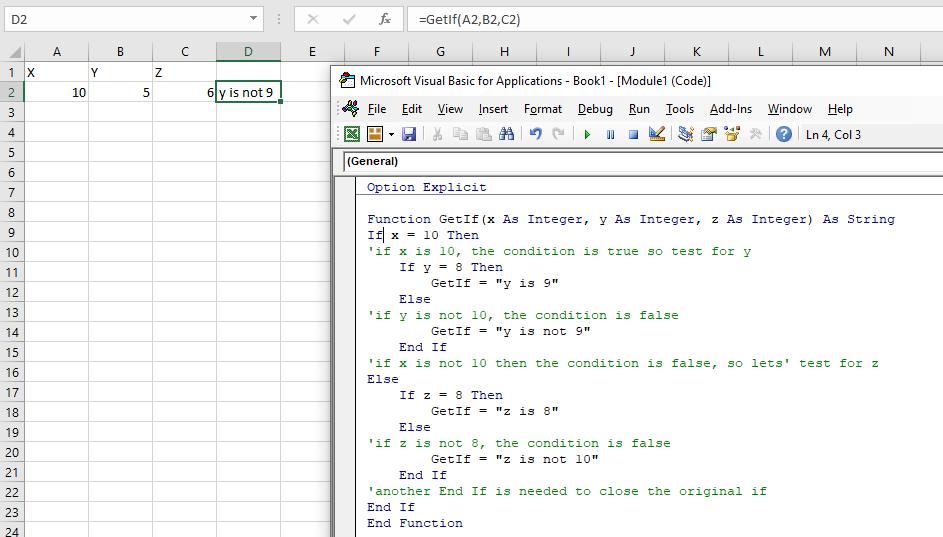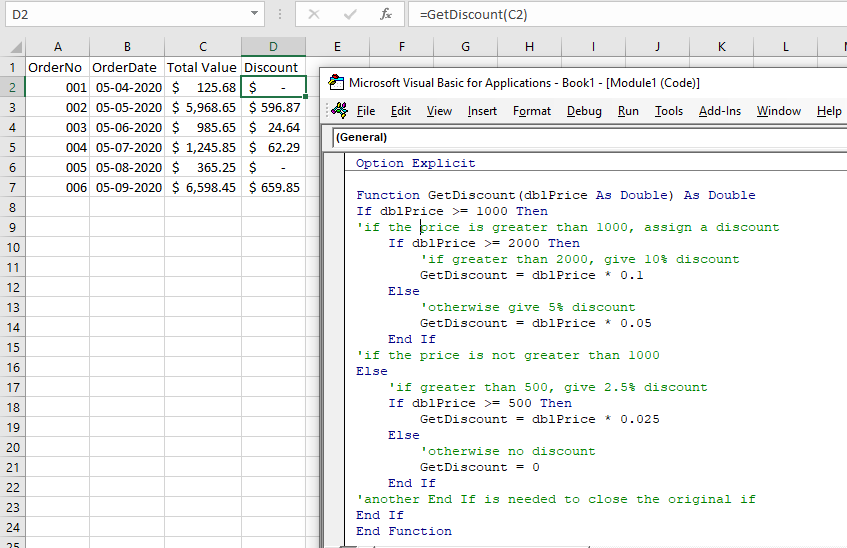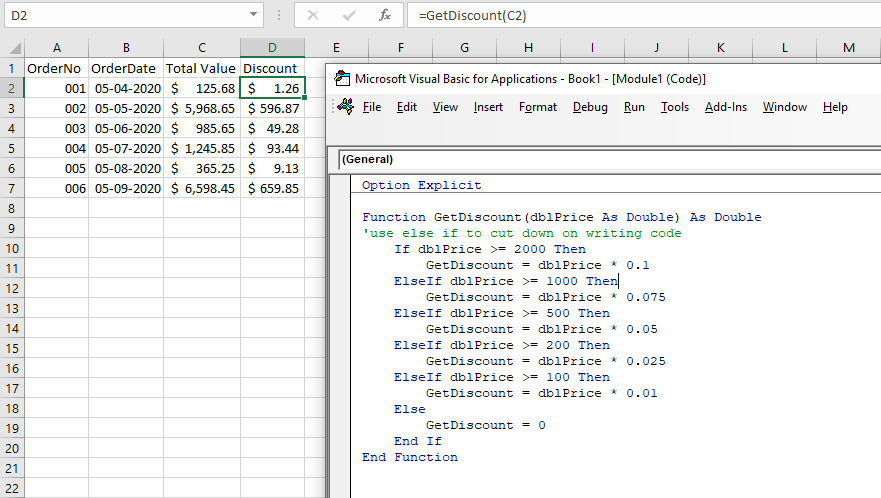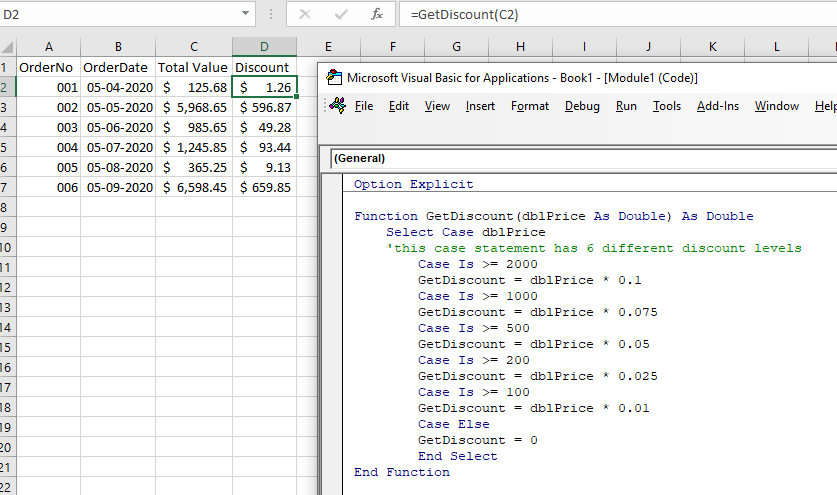# VBA Multiple (Nested) If Statements

This tutorial will show you how to use nested If statements in VBA.

If statements allow you to test for a single condition in VBA to see if the condition is True or False, and depending on the answer, the code will move in the direction of the true statement or the false statement.

## A Single IF statement

``````Sub TestIf
Dim x as Integer
x = 10
If x = 10 then
'if x is 10, the condition is true
MsgBox x is 10"
Else
'if x is not 10, the condition is false
Msgbox "x is not 10"
End If
End Sub``````

## Nested IFs Explained

A Nested If allows you to put multiple conditions INSIDE each of the True and/or False statements of the original If.

``````Sub TestNestedIf()
Dim x as Integer
Dim y as Integer
Dim z as Integer
x = 10
y = 9
z = 8
If x = 10 Then
'if x is 10, the condition is true so test for y
If y = 9 Then
MsgBox "y is 9"
Else
'if y is not 9, the condition is false
Msgbox "y is not 9"
End If
Else
'if x is not 10 then the condition is false, so lets' test for z
If z = 8 Then
MsgBox "z is 8"
Else
'if z is not 8, the condition is false
Msgbox "z is not 8"
End If
'another End If is needed to close the original if
End If
End Sub``````

Indenting your code when you write it always good practice as it makes the code easy to read and follow when you have to come back to it at some stage, or when another programmer has to read it.

We could also create a user designed function (UDF) and call the values of some cells from Excel into the function using parameters.

``````Function GetIf(x as Integer, y as Integer, z as Integer) as String
If x = 10 Then
'if x is 10, the condition is true so test for y
If y = 9 Then
GetIf= "y is 9"
Else
'if y is not 9, the condition is false
GetIf="y is not 9"
End If
Else
'if x is not 10 then the condition is false, so lets' test for z
If z = 8 then
GetIf="z is 8"
Else
'if z is not 8, the condition is false
GetIf="z is not 8"
End If
'another End If is needed to close the original if
End If
End Function``````## Nested If Practical Example

Consider the following function:

``````Function GetDiscount(dblPrice As Double) As Double
If dblPrice >= 1000 Then
'if the price is greater than 1000, assign a discount
If dblPrice >= 2000 Then
'if greater than 2000, give 10% discount
GetDiscount = dblPrice * 0.1
Else
'otherwise give 5% discount
GetDiscount = dblPrice * 0.05
End If
'if the price is not greater than 1000
Else
'if greater than 500, give 2.5% discount
If dblPrice >= 500 Then
GetDiscount = dblPrice * 0.025
Else
'otherwise no discount
GetDiscount = 0
End If
'another End If is needed to close the original if
End If
End Function``````

Using this Function in an Excel sheet, we can test to see the total price for an order, and apply different discounts depending on that total.## Using ElseIf

ElseIf enables us to simplify your code as it only moved down to the second if statement if the first one returns a false.

``````Function GetDiscount(dblPrice As Double) As Double
'use else if to cut down on writing code
If dblPrice >= 2000 Then
GetDiscount = dblPrice * 0.1
ElseIf dblPrice >= 1000 Then
GetDiscount = dblPrice * 0.075
ElseIf dblPrice >= 500 Then
GetDiscount = dblPrice * 0.05
ElseIf dblPrice >= 200 Then
GetDiscount = dblPrice * 0.025
ElseIf dblPrice >= 100 Then
GetDiscount = dblPrice * 0.01
Else
GetDiscount = 0
End If
End Function``````## Using a Case Statement

We can also use a Case Statement to achieve the same effect.

``````Function GetDiscount(dblPrice As Double) As Double
Select Case dblPrice
'this case statement has 6 different discount levels
Case Is >= 2000
GetDiscount = dblPrice * 0.1
Case Is >= 1000
GetDiscount = dblPrice * 0.075
Case Is >= 500
GetDiscount = dblPrice * 0.05
Case Is >= 200
GetDiscount = dblPrice * 0.025
Case Is >= 100
GetDiscount = dblPrice * 0.01
Case Else
GetDiscount = 0
End Select
End Function``````Stop searching for VBA code online. Learn more about AutoMacro - A VBA Code Builder that allows beginners to code procedures from scratch with minimal coding knowledge and with many time-saving features for all users!